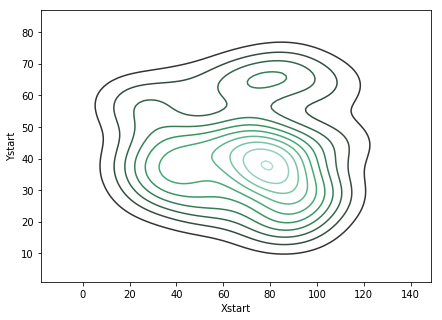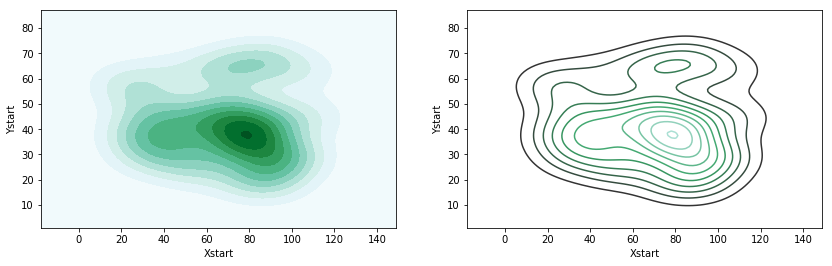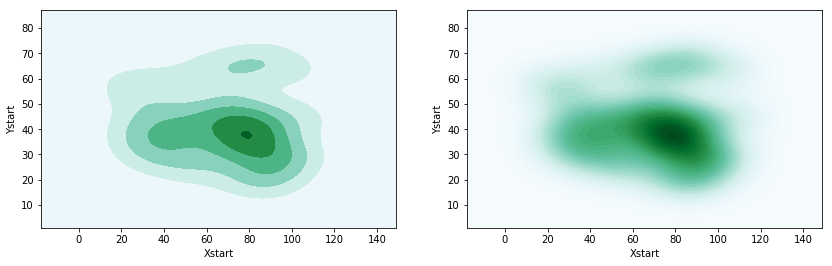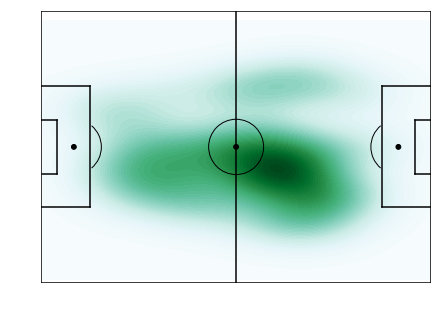# Football Heatmaps with Seaborn

Football heatmaps are used by in-club and media analysts to illustrate the area within which a player has been present. They might illustrate player location, or the events of a player or team and are effectively a smoothed out scatter plot of these points. While there may be some debate as to how much they are useful (they don’t tell you if actions/movement are a good or bad thing!), they can often be very aesthetically pleasing and engaging, hence their popularity. This article will take you through loading your dataset and plotting a heatmap around x & y coordinates in Python.

Let’s get our modules imported and our data ready to go!

In :
```import pandas as pd
import numpy as np
import matplotlib.pyplot as plt
from matplotlib.patches import Arc
import seaborn as sns

%matplotlib inline

```
Out:
Half Time Event Player Team Xstart Ystart Xend Yend
0 First Half 1 Pass Wombech USA 26 38 66 52
1 First Half 6 Pass Wombech USA 81 34 62 68
2 First Half 6 Pass Wombech USA 46 45 84 63
3 First Half 8 Pass Wombech USA 89 66 89 39
4 First Half 9 Pass Wombech USA 68 64 21 25

### Plotting a heatmap

Today’s dataset showcases Wombech’s passes from her match. As you can see, we have time, player and location data. We will be looking to plot the starting X/Y coordinates of Wombech’s passes, but you would be able to do the same with any coordinates that you have – whether GPS/optical tracking coordinates, or other event data.

Python’s Seaborn module makes plotting a tidy dataset incredibly easy with ‘.kdeplot()’. Plotting it with simply the x and y coordinate columns as arguments will give you something like this:

In :
```fig, ax = plt.subplots()
fig.set_size_inches(7, 5)

sns.kdeplot(data["Xstart"],data["Ystart"])
plt.show()
```Cool, we have a contour plot, which groups lines closer to eachother where we have more density in our data.

Let’s customise this with a couple of additional arguments:

• shade: fills the gaps between the lines to give us more of the heatmap effect that we are looking for.
• n_levels: draws more lines – adding lots of these will blur the lines into a heatmap

Take a look at the examples below to see the differences these two arguments produce:

In :
```fig, ax = plt.subplots()
fig.set_size_inches(14,4)

plt.subplot(121)

#Plot two - no shade, lines only
plt.subplot(122)
sns.kdeplot(data["Xstart"],data["Ystart"])

plt.show()
```In :
```fig, ax = plt.subplots()
fig.set_size_inches(14,4)

#Plot One - distinct areas with few lines
plt.subplot(121)

#Plot Two - fade lines with more of them
plt.subplot(122)

plt.show()
```Now that we can customise our plot as we see fit, we just need to add our pitch map. Learn more about plotting pitches here, but feel free to use this pitch map below – although you may need to change the coordinates to fit your data!

Also take note of our xlim and ylim lines – we use these to set the size of the plot, so that the heatmap does not spill over the pitch.

In :
```#Create figure
fig=plt.figure()
fig.set_size_inches(7, 5)

#Pitch Outline & Centre Line
plt.plot([0,0],[0,90], color="black")
plt.plot([0,130],[90,90], color="black")
plt.plot([130,130],[90,0], color="black")
plt.plot([130,0],[0,0], color="black")
plt.plot([65,65],[0,90], color="black")

#Left Penalty Area
plt.plot([16.5,16.5],[65,25],color="black")
plt.plot([0,16.5],[65,65],color="black")
plt.plot([16.5,0],[25,25],color="black")

#Right Penalty Area
plt.plot([130,113.5],[65,65],color="black")
plt.plot([113.5,113.5],[65,25],color="black")
plt.plot([113.5,130],[25,25],color="black")

#Left 6-yard Box
plt.plot([0,5.5],[54,54],color="black")
plt.plot([5.5,5.5],[54,36],color="black")
plt.plot([5.5,0.5],[36,36],color="black")

#Right 6-yard Box
plt.plot([130,124.5],[54,54],color="black")
plt.plot([124.5,124.5],[54,36],color="black")
plt.plot([124.5,130],[36,36],color="black")

#Prepare Circles
centreCircle = plt.Circle((65,45),9.15,color="black",fill=False)
centreSpot = plt.Circle((65,45),0.8,color="black")
leftPenSpot = plt.Circle((11,45),0.8,color="black")
rightPenSpot = plt.Circle((119,45),0.8,color="black")

#Draw Circles

#Prepare Arcs
leftArc = Arc((11,45),height=18.3,width=18.3,angle=0,theta1=310,theta2=50,color="black")
rightArc = Arc((119,45),height=18.3,width=18.3,angle=0,theta1=130,theta2=230,color="black")

#Draw Arcs

#Tidy Axes
plt.axis('off')

plt.ylim(0, 90)
plt.xlim(0, 130)

#Display Pitch
plt.show()
```Great work, now we can see Wombech’s pass locations as a heatmap!

### Summary

Seaborn makes heatmaps a breeze – we simply use the contour plots with ‘kdeplot()’ and blur our lines to give a heatmap effect.

If using these to communicate rather than analyse, always take care. There is nothing telling you if the actions in the plot are good or bad, but we may make these inferences when discussing them. As always, be sure that what you think is being communicated is actually being communicated!

As for next steps, why not take a look at pass maps, or other parts of our visualisation series?